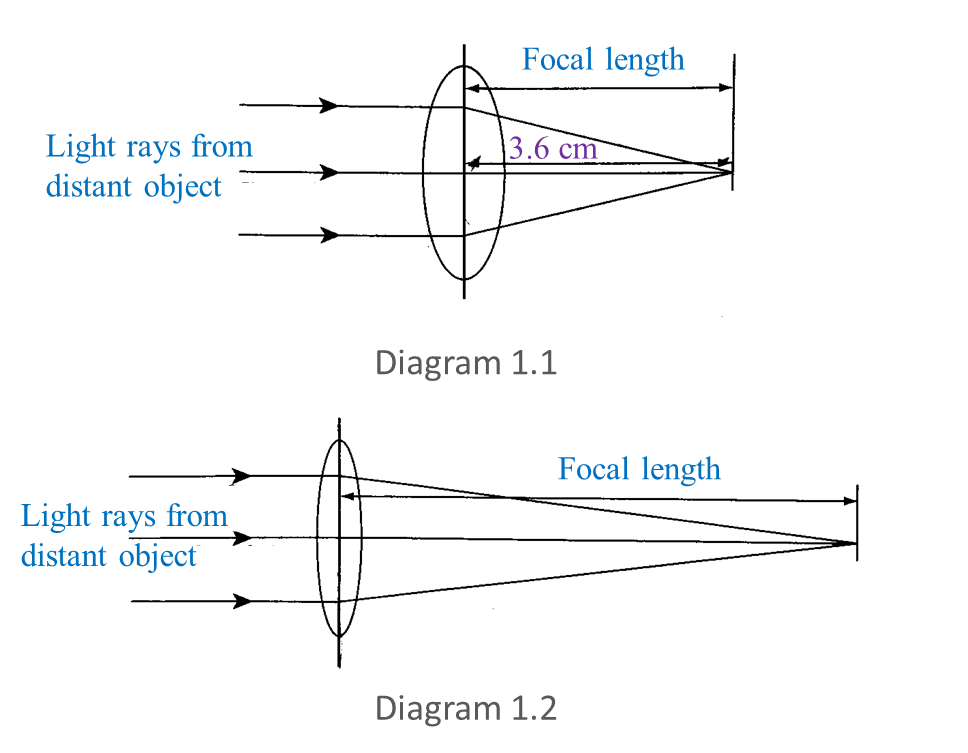# Formation of Images by Lenses (Structured Questions)

Question 1:
Diagram 1.1 and Diagram 1.2 show an experiment to measure the focal length of two convex lenses.(a) Measure and write down the focal length in Diagram 1.1. [1 mark]

(b) Observe Diagram 1.1 and 1.2.
What is the difference between the focal lengths of the two convex lenses? [1 mark]

(c) State one inference for this experiment. [1 mark]

(d) State the variables in this experiment.
(i) Constant variable [1 mark]
(ii) Manipulated variable [1 mark]